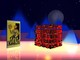## SATOR AREPO TENET OPERAThe Magic Square

## Wednesday, 23 May 2012

### 4 6 3 8 A B K 2 4 A L G M O R 3 Y X 24 89 R P S T O V A LWilliam Blakes 418

The Ending of Hiding in Hadit; and Blessing and Worship to The Prophet of The Lovely Star

We are told in II:75 to "listen to the numbers & the words": the Hebrew for "numbers", SPRVTh, suggests that the numbers of the puzzle relate to the Sephiroth. Therefore, we take the first four numbers as indicating the Sephiroth: ChSD, ThPARTh, BINH, and HVD.

Taking the initials (that is, forming a notariqon), we have Ch+Th+B+H = 415. The closeness to the "magick" number 418 makes it inevitable to add the Hebrew values of the next two letters, AB. Thus, the first six symbols can be made to give the value 418.

Now we take the next eight symbols: K 2 4 A L G M O, and attempt a similar derivation. Take K in full as KP = 100. The numbers 2 and 4 are again read as Sephiroth: ChKMH = 73 and ChSD = 72. "A" is read as an Atu number, and is thus Zero. L is read in full as LMD = 74. G is 3. M is MIM = 90. And the "O" is read as a Vau = 6. Hence, 100 + 73 + 72 + 0 + 74 + 3 + 90 + 6 = 418.

Repeating the pattern of six symbols and then eight: we take R 3 Y X 24 89. R is 200. Reading "3" as BINH, the initial gives us 2. Taking "Y" as Yod yields 10. The "X" presents difficulties, as it is not clear how to treat it as a Hebrew letter: instead, the sign in the manuscript is treated as a skewed "+" sign. "24" and "89" cannot be treated as Sephirotic numbers, being too large: instead, they are treated as Paths between the relevant Sephiroth. Thus "24" is the Path between Chokmah And Chesed, which is Vau = 6. And "89" is the Path between Hod and Yesod, which is Resh = 200. This gives: 200 + 2 + 10 "plus" 6 + 200 = 418.

Of course, the remaining eight symbols have a known solution provided by Crowley: reading Shin and Teth as Atu numbers gives us the familiar RPShTOVAL = 418.

Thus the puzzle yields a fourfold repetition of the number 418. Is there any textual warrant for such a pattern? Perhaps. AL I:51 tells us "There are four gates to one palace ...", and we find in Liber DCCCXIII 2:11 "For mine was the keyword to the Closed Palace 418 ...". Taken together, these suggest a fourfold use of the word/number 418, which the above derivation shows to be potentially implicit in the Puzzle of the numbers & the words.

Of course, this derivation, with its arbitrary use of qabalistic mechanisms, lacks the clarity to be expected of a true solution: but it is an interesting exercise (I hope) in seeing how a meaningful pattern can be abstracted from the semantic "noise" of the perennial enigma of II:76.

☽✪☾Sgian-dubh I won't let them Immanentize the eschaton
En Ferus Hostis I Tego Arcana

I Tego Arcana Dei